RS Aggarwal Class 8 Solutions Chapter 20 - Volume And Surface Area Of Solids

RS Aggarwal Class 8 Chapter 20 - Volume And Surface Area Of Solids Solutions Free PDF

Volume and surface area is one of the major topics of mathematics. In this chapter, the students will learn how to calculate the surface area and volume of solid materials. To master the concepts of volume and surface area of solids students can solve the questions from RS Aggarwal for class 8. To help students solve all the questions and to score good marks in the exam we have provided the RS Aggarwal class 8 solutions chapter 20 volume and surface area of solids.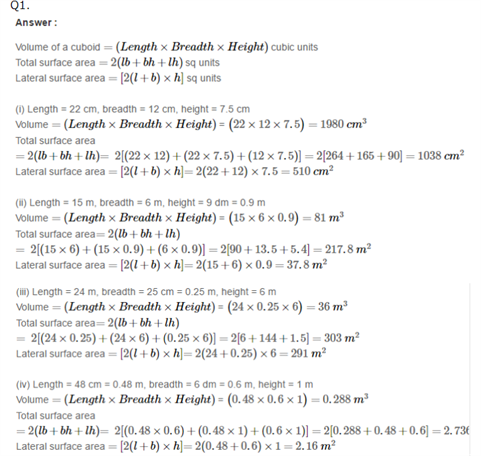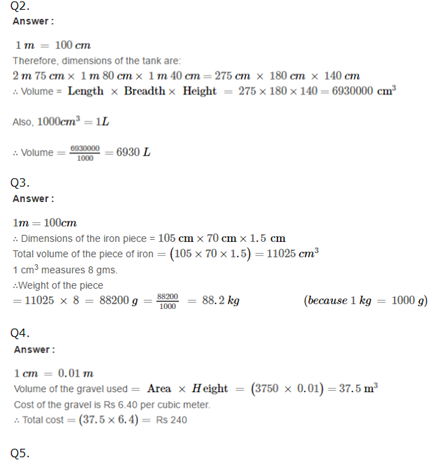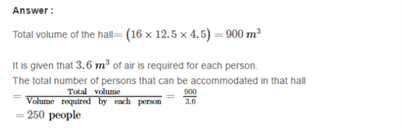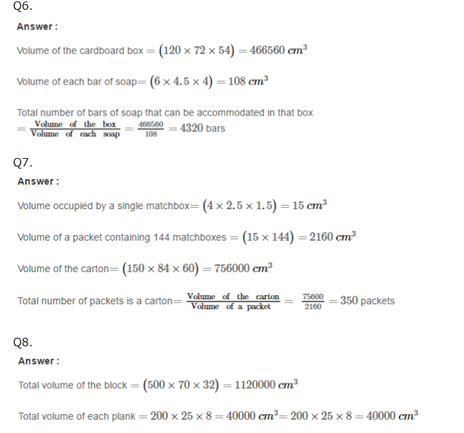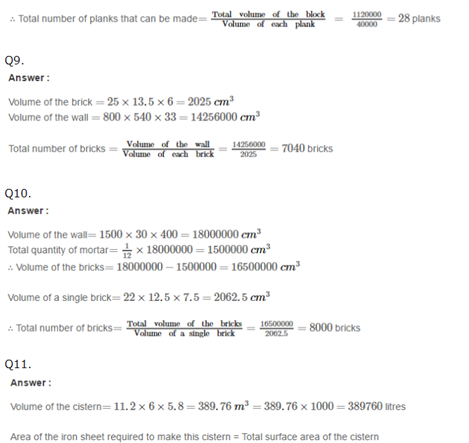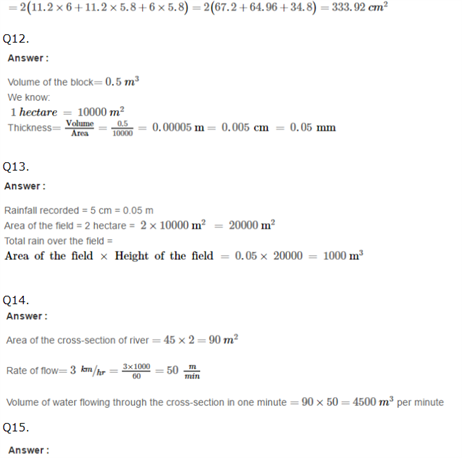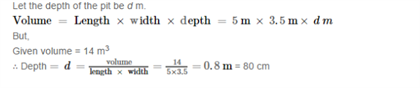Practise This Question

What is the product of (a + b) and 7a2b2?